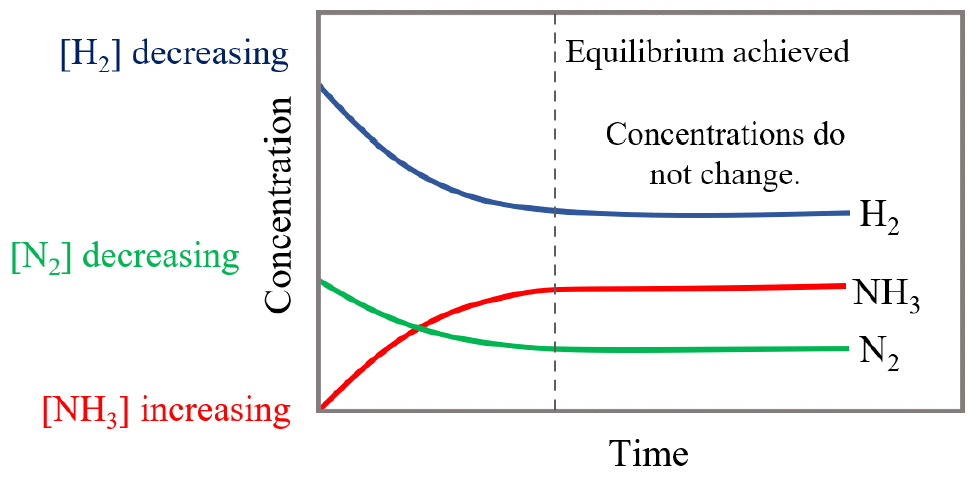## General Chemistry

Some chemical reactions are reversible, meaning they proceed in both forward and reverse directions. These reactions are shown with double arrows:

aA + bB ⇆ cC + dD

When the rates of forward and reverse reactions are equal, the system is said to be at equilibrium. For example, the synthesis of ammonia (NH3) from hydrogen and nitrogen gases carried out at high pressure and temperature in the presence of a metal catalyst known as the Haber process, is one of the most common reactions that establishes an equilibrium:

3 H2(g) + N2(g) ⇆ 2 NH3

Because the reaction proceeds in both directions, it is ambiguous to name the components as reactants and products. However, it is accepted to use the term reactants for the substances on the left side of the chemical equation and the term products for the substances on the right side of the equation.

Let’s see how the concentrations of the reactants and the product change over time.

When the nitrogen and hydrogen are mixed, there is no ammonia in the mixture, and therefore, its concentration increases with time while the concentration of N2 and H2 are decreasing:The dotted line shows the point when the concentrations of reactants and products do not change because the rates of the forward and reverse reactions are equal, and this is when the equilibrium is reached.

Notice the difference in the concentration change (the rate of depletion) of hydrogen and nitrogen gases. Because the stoichiometry in the balanced equation dictates that for every 1 molecule of N2, 3 molecules of H2 are needed, the H2 concentration initially decreases more rapidly as compared to the initial decrease in N2 concentration.

Likewise, the NH3 plot initially increases faster than the N2 plot decreases since, here as well, the ratio of these two gases is 2 : 1 meaning there is 2 times more ammonia produced than nitrogen is consumed at any time of reaction.

The term dynamic equilibrium/state is often used which indicates that the forward and reverse reactions are still occurring. So, the concentrations stay constant not because the reactions in both directions stopped but because they occur at the same rate. All chemical equilibria are dynamic equilibria. Dynamic equilibria respond to addition of even small amounts of components as well as changes in temperature and pressure. This is what distinguishes a dynamic equilibrium from a simple mixture of gases that are not reacting and do not respond to these changes.

In the next articles, we will see how chemical equilibria are described quantitively, and how changing the reaction conditions affect them.

Check Also

1686023504